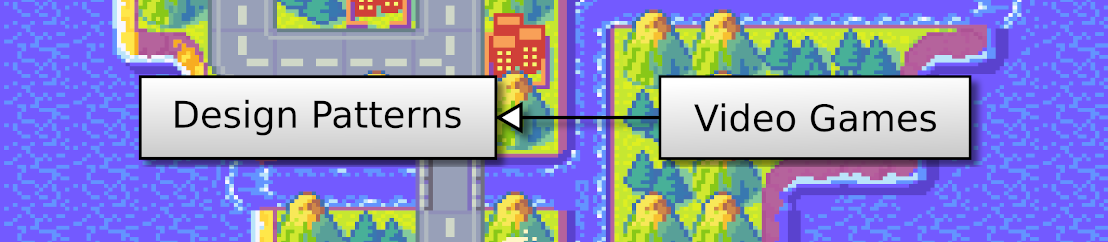## Discover Python and Patterns (16): Inheritance

In this post, I present the basics of class inheritance, to represent our units (the tank and the canon tower) more efficiently.

This post is part of the Discover Python and Patterns series

## Class

A class allows us to "bundle" data in attributes and processing in methods. For instance, let's create a class that stores two integers and a has a single method that returns their sum:

We can implement this in the following way:

``````class Sum:
def __init__(self,x,y):
self.x = x
self.y = y
def compute(self):
return self.x + self.y``````

Let's also create a second class that stores two integers and compute their product. The graphical representation is the same, except for the name of the class:

The implementation is also almost the same, except for the body of the `compute()` method:

``````class Product:
def __init__(self,x,y):
self.x = x
self.y = y
def compute(self):
return self.x * self.y``````

To create this second class, we had to copy half of the first class. In this example, there are few lines, but it many cases, there is a lot of common parts.

## Class inheritance

Class inheritance allows gathering the shared code in a base class, and then to create specific implementations in child classes. For instance, for the case of computation classes:

Using Python, we can implement it in the following way:

``````class Computation:
def __init__(self,x,y):
self.x = x
self.y = y
def compute(self):
pass

class Sum(Computation):
def compute(self):
return self.x + self.y

class Product(Computation):
def compute(self):
return self.x * self.y``````

As you can see, the creation of a new computation class (like `Sum` or `Product`) is quick and easy. We first create a class with a base class, with the following syntax:

``````class ChildClass(BaseClass):
... class body ...``````

Then, we only need to implement specific methods. Note that it also works for the constructor (the `__init__()`method), which means that we can add new attributes in the child classes.

## Method call

The cherry on the cake of class inheritance is that we can call a method in the base class without worrying about its implementation:

``````computations = [ Sum(3,2), Product(4,5) ]
for computation in computations:
print(computation.compute())``````

In this example, the `for` loop in lines 2-3 only needs to know the `Computation` class, and more specifically, that this class has a `compute()` method.

You can add or remove new instances in the `computations`list, including new child classes of `Computation`, lines 2-3 do not need to be updated. Without class inheritance, we would have to add much more code and have to update this code for every new computation class. I hope that you see the incredible time-saving!

## Game units

Now I propose to use this class inheritance to represent our units better:

The `Unit` base class creates attributes: a reference to the game state (to access information like the world size), the location of the unit and the tile in the tileset (for rendering):

``````class Unit():
def __init__(self,state,position,tile):
self.state = state
self.position = position
self.tile = tile
def move(self,moveVector):
raise NotImplementedError()``````

The `move()` method raises a `NotImplementedError` exception. So, if we forget to implement this method in a child class, we will know it.

The `Tank` child class implements the `move()` method. This implementation is very similar to what we were doing in the `GameState` class:

``````class Tank(Unit):
def move(self,moveVector):
newPos = self.position + moveVector

if newPos.x < 0 or newPos.x >= self.state.worldSize.x \
or newPos.y < 0 or newPos.y >= self.state.worldSize.y:
return

for unit in self.state.units:
if newPos == unit.position:
return

self.position = newPos ``````

Lines 5-7: the size of the world can be accessed using the `state` attribute.

Lines 9-11: we still avoid any new location with a unit. This part is now more generic since it handles any units, like another tank player of new enemy type.

The `Tower` child class also implements the `move()` method, but does nothing since towers can't move:

``````class Tower(Unit):
def move(self,moveVector):
pass``````

## GameState class

The `GameState` class needs to be a bit updated:

``````class GameState():
def __init__(self):
self.worldSize = Vector2(16,10)
self.units = [
Tank(self,Vector2(5,4),Vector2(1,0)),
Tower(self,Vector2(10,3),Vector2(0,1)),
Tower(self,Vector2(10,5),Vector2(0,1))
]

def update(self,moveTankCommand):
for unit in self.units:
unit.move(moveTankCommand)``````

The constructor creates instances of units instead of 2D vectors (lines 4-8). It is more generic since we could add another kind of unit with more specific properties.

The `update()` method calls the `move()` method of each unit (lines 11-12). If there is only one tank in the `units` list, it will be the only one to be moved by the player. Try to add a second one at a different initial location in the `units`list, and see the two tanks moving when you press the arrow keys. You can also try to add many tanks in the list and get a quite complex behavior with a simple code.

Considering the Command pattern, the basic version I presented is not able to handle more complex controls (like two players controlling two different tanks). I need to introduce more concepts to show you a better solution. It will be the subject of a future post.

## UserInterface class

The `UserInterface` class is also very similar, except for the `renderUnit()` method that replaces the previous `renderTower()`method:

``````def renderUnit(self,unit):
# Location on screen
spritePoint = unit.position.elementwise()*self.cellSize

# Unit texture
texturePoint = unit.tile.elementwise()*self.cellSize
textureRect = Rect(int(texturePoint.x), int(texturePoint.y), int(self.cellSize.x),int(self.cellSize.y))
self.window.blit(self.unitsTexture,spritePoint,textureRect)

# Weapon texure
texturePoint = Vector2(0,6).elementwise()*self.cellSize
textureRect = Rect(int(texturePoint.x), int(texturePoint.y), int(self.cellSize.x),int(self.cellSize.y))
self.window.blit(self.unitsTexture,spritePoint,textureRect)

def render(self):
self.window.fill((0,0,0))

# Towers
for unit in self.gameState.units:
self.renderUnit(unit)

pygame.display.update()    ``````

We now have a single rendering block. Each case is rendering in a specific location (`unit.position`) and a specific tile (`unit.tile`).

Note that we could also use class inheritance to specialize the rendering of each unit.